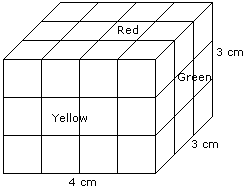# Verbal Reasoning - Cube and Cuboid

Exercise : Cube and Cuboid - Cube and Cuboid 2
Directions to Solve

The following questions are based on the information given below:

1. There is a cuboid whose dimensions are 4 x 3 x 3 cm.
2. The opposite faces of dimensions 4 x 3 are coloured yellow.
3. The opposite faces of other dimensions 4 x 3 are coloured red.
4. The opposite faces of dimensions 3 x 3 are coloured green.
5. Now the cuboid is cut into small cubes of side 1 cm.

1.
How many small cubes will have only two faces coloured ?
12
24
16
12
Explanation:Number of small cubes having only two faces coloured = 6 from the front + 6 from the back + 2 from the left + 2 from the right

= 16

2.
How many small cubes have three faces coloured ?
24
20
16
8
Explanation:Such cubes are related to the corners of the cuboid and there are 8 corners.

Hence, the required number is 8.

3.
How many small cubes will have no face coloured ?
1
2
4
8
Explanation:Number of small cubes have no face coloured = (4 - 2) x (3 - 2) = 2 x 1 = 2

4.
How many small cubes will have only one face coloured ?
10
12
14
18